The total mechanical energy of a system is conserved if the forces, doing work on it, are conservative.

Suppose that a body undergoes displacement Δx under the action of a conservative force F. Then from the WE theorem we have,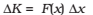If the force is conservative, the potential energy function V(x) can be defined such that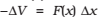The above equations imply that which means that K + V, the sum of the kinetic and potential energies of the body is constant.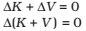Over the whole path, xi to xf, this means that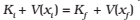The quantity K +V(x), is called the total mechanical energy of the system. Individually the kinetic energy K and the potential energy V(x) may vary from point to point, but the sum is a constant.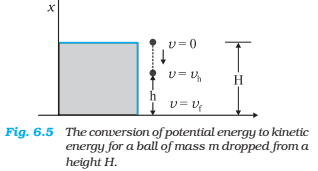The total mechanical energies E0, Eh, and EH of the ball at the indicated heights zero (ground level), h and H, are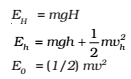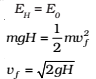a result that was obtained for a freely falling body.
Further,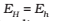which implies,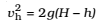and is a familiar result from kinematics.

At the height H, the energy is purely potential. It is partially covered to kinetic at height h and is fully kinetic at ground level. This illustrates the conservation of mechanical energy.